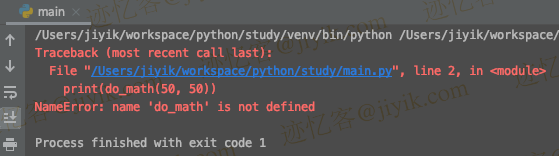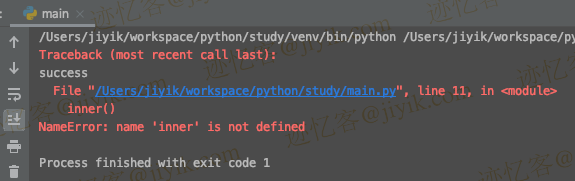# 迹忆客专注技术分享

## Python NameError: function is not defined 错误

``````# ⛔️ NameError: name 'do_math' is not defined
print(do_math(50, 50))

def do_math(a, b):
return a + b
````````````def do_math(a, b):
return a + b

print(do_math(50, 50))  # 👉️ 100
``````

Python "NameError: function is not defined" 的出现有多种原因：

1. 调用不存在的函数。
2. 在声明函数之前调用它。
3. 函数名称拼写错误（名称区分大小写）。
4. 使用内置模块而不先导入它们。
5. 从外部调用作用域函数。 例如，声明一个嵌套函数并尝试从外部调用它。

``````def do_math(a, b):
return a + b

# ⛔️ NameError: name 'do_Math' is not defined. Did you mean: 'do_math'?
print(do_Math(50, 50))  # 👈️ function name misspelled
````````````# ⛔️ NameError: name 'math' is not defined
print(math.floor(17.7))
``````

``````import math

print(math.floor(17.7))  # 👉️ 17
``````

``````def outer():
def inner():
return 'hello world'

print('success')

outer()

# ⛔️ NameError: name 'inner' is not defined. Did you mean: 'iter'?
inner()
```````inner` 函数是在 `outer` 函数内部声明的，所以我们不能从外部范围调用它。

``````def inner():
return 'hello world'

def outer():
print(inner())
print('success')

outer()

inner()
``````

``````def outer():
def inner():
return 'hello world'

result = inner()
return result

print(outer())  # 👉️ "hello world"
``````

## 相关文章

### 在 Python 中创建临时文件## 热门标签